# jQuery UI | Tabs

• Last Updated : 05 Dec, 2019

Tabs are used to create multiple sections on a webpage that can be swapped, much like an accordion. It helps to group content and to view content from a specific group at a time.

Tabs are created by following a specific markup which is as follows:

• Tabs should be enclosed inside an ordered list or an unordered list
• Each tab heading should be wrapped individually in a list item and an anchored tag enclosed with an href attribute specifying the content for each tab panel
• Each tab panel can be empty but it should have its own id corresponding to the hashed name entered in the anchor element of the associated tab.

The contents inside a tab panel can be defined on the same page or can be loaded through Ajax, both of them are handled by the href attribute associated with the anchor tag of that panel.
Below we write a code for a simple 4-panel tab using jquery UI.

The tabs are specified in a div tag with an id. The id of which is specified inside the jquery code. Here we have chosen the 2nd tab as the default tab which will be chosen when the webpage opens. You can change it by specifying a different value in the active option.
Note: The tabs are indexed starting from “0”.

Below examples illustrates the jQuery Tabs:
Example 1: This example code is a simple jquery tab, all the tabs are assessable.

• Program:

 `<``html``>`` ` `<``head``>``    ``<``link` `href``=``'https://ajax.googleapis.com/ajax/libs/jqueryui/1.12.1/themes/ui-darkness/jquery-ui.css'` `          ``rel``=``'stylesheet'``>````    ``<``style``>``        ``b{``            ``float: right;``            ``margin: 7px;``            ``color: lime;``        ``}``         ` `        ``#geeks a:hover{``            ``color: lime;``            ``background: gray;``        ``}``         ` `    ````<``body``>``    ``<``br``>``    ``<``br``>``    ``<``div` `id``=``"geeks"``>``        ``<``ul``>`` ` `            ``<``li``><``a` `href``=``"#Algorithms"``>Algorithms``            ``<``li``><``a` `href``=``"#Data_Structure"``>Data Structure``            ``<``li``><``a` `href``=``"#Practice"``>Practice``            ``<``li``><``a` `href``=``"#interview"``>interview``             ` `            ``<``b``>GeeksforGeeks``        ```` ` `        ``<``div` `id``=``'Algorithms'``>``            ``<``p``>``                ``The answer to this is simple, we can have all the ``                ``above things only if we have performance. So ``                ``performance is like currency through which we can``                ``buy all the above things. Another reason ``                ``for studying performance is – speed is fun!``            ````        ```` ` `        ``<``div` `id``=``'Data_Structure'``>``            ``<``p``>``                ``For example, let us say, we want to store marks of ``                ``all students in a class, we can use an array to store``                ``them. This helps in reducing the use of number of ``                ``variables as we don’t need to create a separate ``                ``variable for marks of every subject.  All marks can``                ``be accessed by simply traversing the array.``            ````        ```` ` ` ` `        ``<``div` `id``=``'Practice'``>``            ``<``p``>``                ``Asymptotic Analysis is the big idea that handles ``                ``above issues in analyzing algorithms. In Asymptotic``                ``Analysis, we evaluate the performance of an algorithm``                ``in terms of input size (we don’t measure the actual ``                ``running time). We calculate, how does the time ``                ``(or space) taken by an algorithm increases with ``                ``the input size.``            ``    ``        ```` ` `        ``<``div` `id``=``'interview'``>``            ``<``p``>``                ``Also, in Asymptotic analysis, we always talk about ``                ``input sizes larger than a constant value. It might ``                ``be possible that those large inputs are never given``                ``to your software and an algorithm  which is ``                ``asymptotically slower, always performs better for ``                ``your particular situation. So, you may end up choosing``                ``an algorithm that is Asymptotically slower but faster ``                ``for your software.``            ````        ```` ` `    ````    ``<``script` `src``=``"https://ajax.googleapis.com/ajax/libs/jquery/3.1.1/jquery.min.js"``>``    ````    ``<``script` `src``=``"https://ajax.googleapis.com/ajax/libs/jqueryui/1.12.1/jquery-ui.min.js"``>``    ````    ``<``script``>``        ``\$(document).ready(function() {``            ``\$("#geeks").tabs({``                    ``active: 0``                ``})``        ``})``    ```` ` ``` ` ``

• Output: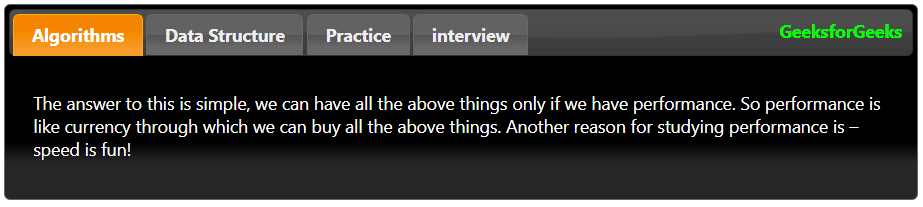Example 2: Keeping all tabs closed by default, you can also choose to keep all the tabs closed by default. To do this we use the Collapsible option and set its value to “True” and set the value of active option to false.

• Program:

 `<``html``>`` ` `<``head``>``    ``<``link` `href``=``'https://ajax.googleapis.com/ajax/libs/jqueryui/1.12.1/themes/ui-darkness/jquery-ui.css'` `          ``rel``=``'stylesheet'``>````    ``<``style``>``        ``b{``            ``float: right;``            ``margin: 7px;``            ``color: lime;``        ``}``         ` `        ``#geeks a:hover{``            ``color: lime;``            ``background: gray;``        ``}``         ` `    ````<``body``>``    ``<``br``>``    ``<``br``>``    ``<``div` `id``=``"geeks"``>``        ``<``ul``>`` ` `            ``<``li``><``a` `href``=``"#Algorithms"``>Algorithms``            ``<``li``><``a` `href``=``"#Data_Structure"``>Data Structure``            ``<``li``><``a` `href``=``"#Practice"``>Practice``            ``<``li``><``a` `href``=``"#interview"``>interview``             ` `            ``<``b``>GeeksforGeeks``        ```` ` `        ``<``div` `id``=``'Algorithms'``>``            ``<``p``>``                ``The answer to this is simple, we can have all the ``                ``above things only if we have performance. So ``                ``performance is like currency through which we can``                ``buy all the above things. Another reason ``                ``for studying performance is – speed is fun!``            ````        ```` ` `        ``<``div` `id``=``'Data_Structure'``>``            ``<``p``>``                ``For example, let us say, we want to store marks of ``                ``all students in a class, we can use an array to store``                ``them. This helps in reducing the use of number of ``                ``variables as we don’t need to create a separate ``                ``variable for marks of every subject.  All marks can``                ``be accessed by simply traversing the array.``            ````        ```` ` ` ` `        ``<``div` `id``=``'Practice'``>``            ``<``p``>``                ``Asymptotic Analysis is the big idea that handles ``                ``above issues in analyzing algorithms. In Asymptotic``                ``Analysis, we evaluate the performance of an algorithm``                ``in terms of input size (we don’t measure the actual ``                ``running time). We calculate, how does the time ``                ``(or space) taken by an algorithm increases with ``                ``the input size.``            ``    ``        ```` ` `        ``<``div` `id``=``'interview'``>``            ``<``p``>``                ``Also, in Asymptotic analysis, we always talk about ``                ``input sizes larger than a constant value. It might ``                ``be possible that those large inputs are never given``                ``to your software and an algorithm  which is ``                ``asymptotically slower, always performs better for ``                ``your particular situation. So, you may end up choosing``                ``an algorithm that is Asymptotically slower but faster ``                ``for your software.``            ````        ```` ` `    ````    ``<``script` `src``=``"https://ajax.googleapis.com/ajax/libs/jquery/3.1.1/jquery.min.js"``>``    ````    ``<``script` `src``=``"https://ajax.googleapis.com/ajax/libs/jqueryui/1.12.1/jquery-ui.min.js"``>``    ````    ``<``script``>``        ``\$(document).ready(function() {``            ``\$("#geeks").tabs({``                    ``active: false,``                    ``collapsible: true``                ``})``        ``})``    ```` ` ``` ` ``

• Output: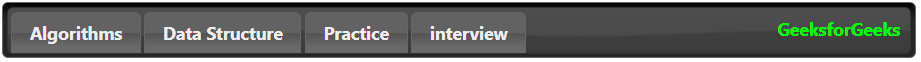Example 3: In this example we will disable the tabs. We can choose to disable specific tabs or all the tabs by using the disable option. First, we disable all the tabs for which we set the value “True” for the disable option.

• Program:

 `<``html``>`` ` `<``head``>``    ``<``link` `href``=``'https://ajax.googleapis.com/ajax/libs/jqueryui/1.12.1/themes/ui-darkness/jquery-ui.css'` `          ``rel``=``'stylesheet'``>````    ``<``style``>``        ``b{``            ``float: right;``            ``margin: 7px;``            ``color: lime;``        ``}``         ` `        ``#geeks a:hover{``            ``color: lime;``            ``background: gray;``        ``}``         ` `    ````<``body``>``    ``<``br``>``    ``<``br``>``    ``<``div` `id``=``"geeks"``>``        ``<``ul``>`` ` `            ``<``li``><``a` `href``=``"#Algorithms"``>Algorithms``            ``<``li``><``a` `href``=``"#Data_Structure"``>Data Structure``            ``<``li``><``a` `href``=``"#Practice"``>Practice``            ``<``li``><``a` `href``=``"#interview"``>interview``             ` `            ``<``b``>GeeksforGeeks``        ```` ` `        ``<``div` `id``=``'Algorithms'``>``            ``<``p``>``                ``The answer to this is simple, we can have all the ``                ``above things only if we have performance. So ``                ``performance is like currency through which we can``                ``buy all the above things. Another reason ``                ``for studying performance is – speed is fun!``            ````        ```` ` `        ``<``div` `id``=``'Data_Structure'``>``            ``<``p``>``                ``For example, let us say, we want to store marks of ``                ``all students in a class, we can use an array to store``                ``them. This helps in reducing the use of number of ``                ``variables as we don’t need to create a separate ``                ``variable for marks of every subject.  All marks can``                ``be accessed by simply traversing the array.``            ````        ```` ` ` ` `        ``<``div` `id``=``'Practice'``>``            ``<``p``>``                ``Asymptotic Analysis is the big idea that handles ``                ``above issues in analyzing algorithms. In Asymptotic``                ``Analysis, we evaluate the performance of an algorithm``                ``in terms of input size (we don’t measure the actual ``                ``running time). We calculate, how does the time ``                ``(or space) taken by an algorithm increases with ``                ``the input size.``            ``    ``        ```` ` `        ``<``div` `id``=``'interview'``>``            ``<``p``>``                ``Also, in Asymptotic analysis, we always talk about ``                ``input sizes larger than a constant value. It might ``                ``be possible that those large inputs are never given``                ``to your software and an algorithm  which is ``                ``asymptotically slower, always performs better for ``                ``your particular situation. So, you may end up choosing``                ``an algorithm that is Asymptotically slower but faster ``                ``for your software.``            ````        ```` ` ` ` `    ````    ``<``script` `src``=``"https://ajax.googleapis.com/ajax/libs/jquery/3.1.1/jquery.min.js"``>``    ````    ``<``script` `src``=``"https://ajax.googleapis.com/ajax/libs/jqueryui/1.12.1/jquery-ui.min.js"``>``    ````    ``<``script``>``        ``\$(document).ready(function() {`` ` `            ``\$( "#geeks" ).tabs({``                ``disabled:true``            ``})`` ` `        ``})``    ```` ` ```                    `

• Output: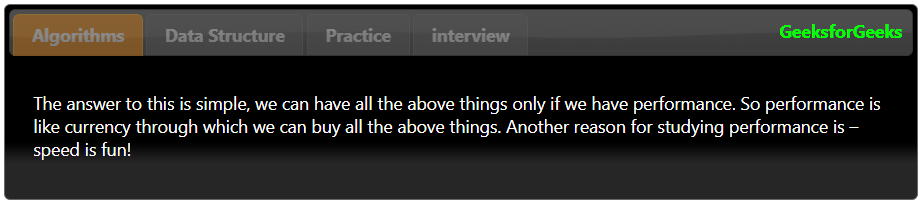Example 4: In this example we will disable some specific tabs. In the below code we disable the 1st and 3rd tab(0 nd 2nd in terms on indexing):

• Program:

 `<``html``>`` ` `<``head``>``    ``<``link` `href``=``'https://ajax.googleapis.com/ajax/libs/jqueryui/1.12.1/themes/ui-darkness/jquery-ui.css'``        ``rel``=``'stylesheet'``>````    ``<``style``>``        ``b{``            ``float: right;``            ``margin: 7px;``            ``color: lime;``        ``}``         ` `        ``#geeks a:hover{``            ``color: lime;``            ``background: gray;``        ``}``         ` `    ````<``body``>``    ``<``br``>``    ``<``br``>``    ``<``div` `id``=``"geeks"``>``        ``<``ul``>`` ` `            ``<``li``><``a` `href``=``"#Algorithms"``>Algorithms``            ``<``li``><``a` `href``=``"#Data_Structure"``>Data Structure``            ``<``li``><``a` `href``=``"#Practice"``>Practice``            ``<``li``><``a` `href``=``"#interview"``>interview``             ` `            ``<``b``>GeeksforGeeks``        ```` ` `        ``<``div` `id``=``'Algorithms'``>``            ``<``p``>``                ``The answer to this is simple, we can have all the ``                ``above things only if we have performance. So ``                ``performance is like currency through which we can``                ``buy all the above things. Another reason ``                ``for studying performance is – speed is fun!``            ````        ```` ` `        ``<``div` `id``=``'Data_Structure'``>``            ``<``p``>``                ``For example, let us say, we want to store marks of ``                ``all students in a class, we can use an array to store``                ``them. This helps in reducing the use of number of ``                ``variables as we don’t need to create a separate ``                ``variable for marks of every subject.  All marks can``                ``be accessed by simply traversing the array.``            ````        ```` ` ` ` `        ``<``div` `id``=``'Practice'``>``            ``<``p``>``                ``Asymptotic Analysis is the big idea that handles ``                ``above issues in analyzing algorithms. In Asymptotic``                ``Analysis, we evaluate the performance of an algorithm``                ``in terms of input size (we don’t measure the actual ``                ``running time). We calculate, how does the time ``                ``(or space) taken by an algorithm increases with ``                ``the input size.``            ``    ``        ```` ` `        ``<``div` `id``=``'interview'``>``            ``<``p``>``                ``Also, in Asymptotic analysis, we always talk about ``                ``input sizes larger than a constant value. It might ``                ``be possible that those large inputs are never given``                ``to your software and an algorithm  which is ``                ``asymptotically slower, always performs better for ``                ``your particular situation. So, you may end up choosing``                ``an algorithm that is Asymptotically slower but faster ``                ``for your software.``            ````        ```` ` ` ` `    ````    ``<``script` `src``=``"https://ajax.googleapis.com/ajax/libs/jquery/3.1.1/jquery.min.js"``>``    ````    ``<``script` `src``=``"https://ajax.googleapis.com/ajax/libs/jqueryui/1.12.1/jquery-ui.min.js"``>``    ````    ``<``script``>``        ``\$(document).ready(function() {`` ` `            ``\$( "#geeks" ).tabs({``                ``active: 1,``                ``disabled:[0, 2]``            ``})`` ` `        ``})``    ```` ` ```                    `

• Output: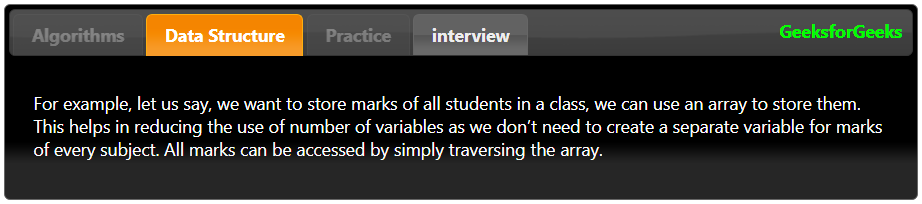Example 5: We can choose which element to open by default, also by default mouse click event opens the tab, also we will change this to Mouse-hover to open or active that tab

• Program:

 `<``html``>``<``head``>``    ``<``link` `href``=``'https://ajax.googleapis.com/ajax/libs/jqueryui/1.12.1/themes/ui-darkness/jquery-ui.css'``        ``rel``=``'stylesheet'``>````    ``<``style``>``        ``b{``            ``float: right;``            ``margin: 7px;``            ``color: lime;``        ``}``         ` `        ``#geeks a:hover{``            ``color: lime;``            ``background: gray;``        ``}``         ` `    ````<``body``>``    ``<``br``>``    ``<``br``>``    ``<``div` `id``=``"geeks"``>``        ``<``ul``>`` ` `            ``<``li``><``a` `href``=``"#Algorithms"``>Algorithms``            ``<``li``><``a` `href``=``"#Data_Structure"``>Data Structure``            ``<``li``><``a` `href``=``"#Practice"``>Practice``            ``<``li``><``a` `href``=``"#interview"``>interview``             ` `            ``<``b``>GeeksforGeeks``        ```` ` `        ``<``div` `id``=``'Algorithms'``>``            ``<``p``>``                ``The answer to this is simple, we can have all the ``                ``above things only if we have performance. So ``                ``performance is like currency through which we can``                ``buy all the above things. Another reason ``                ``for studying performance is – speed is fun!``            ````        ```` ` `        ``<``div` `id``=``'Data_Structure'``>``            ``<``p``>``                ``For example, let us say, we want to store marks of ``                ``all students in a class, we can use an array to store``                ``them. This helps in reducing the use of number of ``                ``variables as we don’t need to create a separate ``                ``variable for marks of every subject.  All marks can``                ``be accessed by simply traversing the array.``            ````        ```` ` ` ` `        ``<``div` `id``=``'Practice'``>``            ``<``p``>``                ``Asymptotic Analysis is the big idea that handles ``                ``above issues in analyzing algorithms. In Asymptotic``                ``Analysis, we evaluate the performance of an algorithm``                ``in terms of input size (we don’t measure the actual ``                ``running time). We calculate, how does the time ``                ``(or space) taken by an algorithm increases with ``                ``the input size.``            ``    ``        ```` ` `        ``<``div` `id``=``'interview'``>``            ``<``p``>``                ``Also, in Asymptotic analysis, we always talk about ``                ``input sizes larger than a constant value. It might ``                ``be possible that those large inputs are never given``                ``to your software and an algorithm  which is ``                ``asymptotically slower, always performs better for ``                ``your particular situation. So, you may end up choosing``                ``an algorithm that is Asymptotically slower but faster ``                ``for your software.``            ````        ```` ` `    ````    ``<``script` `src``=``"https://ajax.googleapis.com/ajax/libs/jquery/3.1.1/jquery.min.js"``>``    ````    ``<``script` `src``=``"https://ajax.googleapis.com/ajax/libs/jqueryui/1.12.1/jquery-ui.min.js"``>``    ````    ``<``script``>``        ``\$(document).ready(function() {`` ` `            ``\$( "#geeks" ).tabs({``                ``event:'mouseover'``            ``})`` ` `        ``})``    ```` ` ```                    `

• Output:Example 6: In this example we will change the tab page height depending on the content of that tab. To do that we will be required to define the min-height as short as you can define. And the overflow property that will increase the height of the tab page depending on the content.

Program:

•  `<``html``>``<``head``>``    ``<``link` `href``=``'https://ajax.googleapis.com/ajax/libs/jqueryui/1.12.1/themes/ui-darkness/jquery-ui.css'``        ``rel``=``'stylesheet'``>````    ``<``style``>``        ``b{``            ``float: right;``            ``margin: 7px;``            ``color: lime;``        ``}``         ` `        ``#geeks a:hover{``            ``color: lime;``            ``background: gray;``        ``}``         ` `    ````<``body``>``    ``<``br``>``    ``<``br``>``    ``<``div` `id``=``"geeks"``>``        ``<``ul``>`` ` `            ``<``li``><``a` `href``=``"#Algorithms"``>Algorithms``            ``<``li``><``a` `href``=``"#Data_Structure"``>Data Structure``            ``<``li``><``a` `href``=``"#Practice"``>Practice``            ``<``li``><``a` `href``=``"#interview"``>interview``             ` `            ``<``b``>GeeksforGeeks``        ```` ` `        ``<``div` `id``=``'Algorithms'``>``            ``<``p``>``                ``The answer to this is simple, we can have all the ``                ``above things only if we have performance. So ``                ``performance is like currency through which we can``                ``buy all the above things. Another reason ``                ``for studying performance is – speed is fun!``            ````        ```` ` `        ``<``div` `id``=``'Data_Structure'``>``            ``<``p``>``                ``For example, let us say, we want to store marks of ``                ``all students in a class, we can use an array to store``                ``them. This helps in reducing the use of number of ``                ``variables as we don’t need to create a separate ``                ``variable for marks of every subject.  All marks can``                ``be accessed by simply traversing the array.``            ````        ```` ` ` ` `        ``<``div` `id``=``'Practice'``>``            ``<``p``>``                ``Asymptotic Analysis is the big idea that handles ``                ``above issues in analyzing algorithms. In Asymptotic``                ``Analysis, we evaluate the performance of an algorithm``                ``in terms of input size (we don’t measure the actual ``                ``running time). We calculate, how does the time ``                ``(or space) taken by an algorithm increases with ``                ``the input size.``            ``    ``        ```` ` `        ``<``div` `id``=``'interview'``>``            ``<``p``>``                ``Also, in Asymptotic analysis, we always talk about ``                ``input sizes larger than a constant value. It might ``                ``be possible that those large inputs are never given``                ``to your software and an algorithm  which is ``                ``asymptotically slower, always performs better for ``                ``your particular situation. So, you may end up choosing``                ``an algorithm that is Asymptotically slower but faster ``                ``for your software.``            ````        ```` ` `    ````    ``<``script` `src``=``"https://ajax.googleapis.com/ajax/libs/jquery/3.1.1/jquery.min.js"``>``    ````    ``<``script` `src``=``"https://ajax.googleapis.com/ajax/libs/jqueryui/1.12.1/jquery-ui.min.js"``>``    ````    ``<``script``>``        ``\$(document).ready(function() {``            ``\$("#geeks").tabs().css({``               ``'min-height': '100px',``               ``'overflow': 'auto'``            ``});``        ``})``    ```` ` ```                    `

• Output: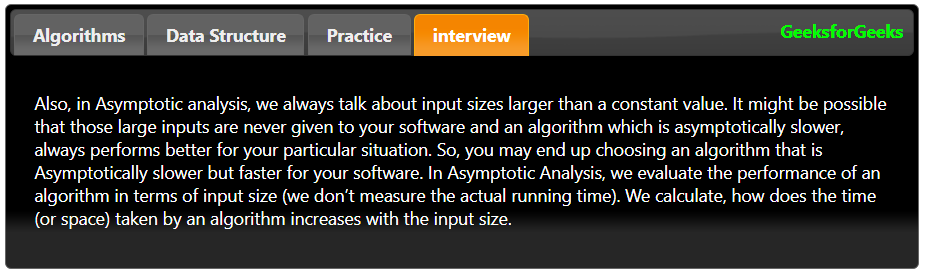My Personal Notes arrow_drop_up